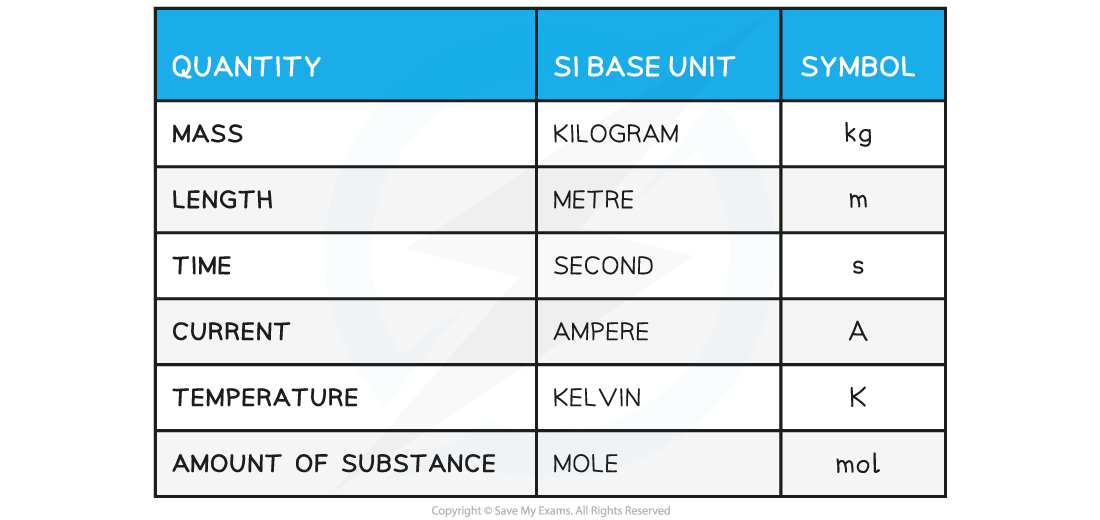# IB DP Physics: SL复习笔记1.1.1 SI Units

### SI Base Quantities

#### International System (S.I.) Units

• There is a seemingly endless number of units in Physics
• These can all be reduced to seven base units from which every other unit can be derived
• These seven units are referred to as the SI Base Units; making up the system of measurement officially used in almost every country around the world

SI Base Quantities TableSix SI quantities are shown. The seventh quantity, the candela, measures luminous intensity, and is not covered in IB Physics. You may meet it later if you continue with Physics at university.

### Derived Units

#### Derived Units

• Derived units are derived from the seven SI Base units
• The base units of physical quantities such as:
• Newtons, N
• Joules, J
• Pascals, Pa, can be deduced
• To deduce the base units, it is necessary to use the definition of the quantity
• The Newton (N), the unit of force, is defined by the equation:
• Force = mass × acceleration
• N = kg × m s–2 = kg m s–2
• Therefore, the Newton (N) in SI base units is kg m s–2
• The Joule (J), the unit of energy, is defined by the equation:
• Energy = ½ × mass × velocity2
• J = kg × (m s–1)2 = kg m2 s–2
• Therefore, the Joule (J) in SI base units is kg m2 s–2
• The Pascal (Pa), the unit of pressure, is defined by the equation:
• Pressure = force ÷ area
• Pa = N ÷ m2 = (kg m s–2) ÷ m2 = kg m–1 s–2
• Therefore, the Pascal (Pa) in SI base units is kg m–1 s–2